Data Structures
Edirlei Soares de Lima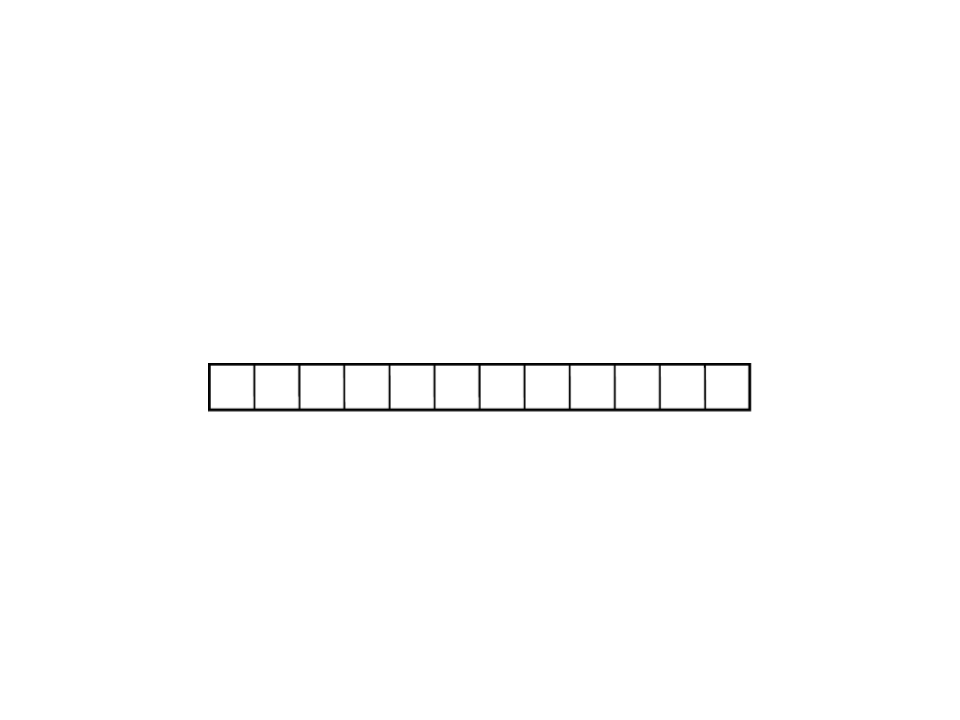Dynamic Structures vs Arrays
Array:
Occupies a continuous space in memory;
Allows random data access;
Requires the predefinition of memory space needed;
Dynamic Structure:
Grow in size (or decrease) as elements are inserted (or removed);
Examples: Linked lists, stacks, queues, trees, ...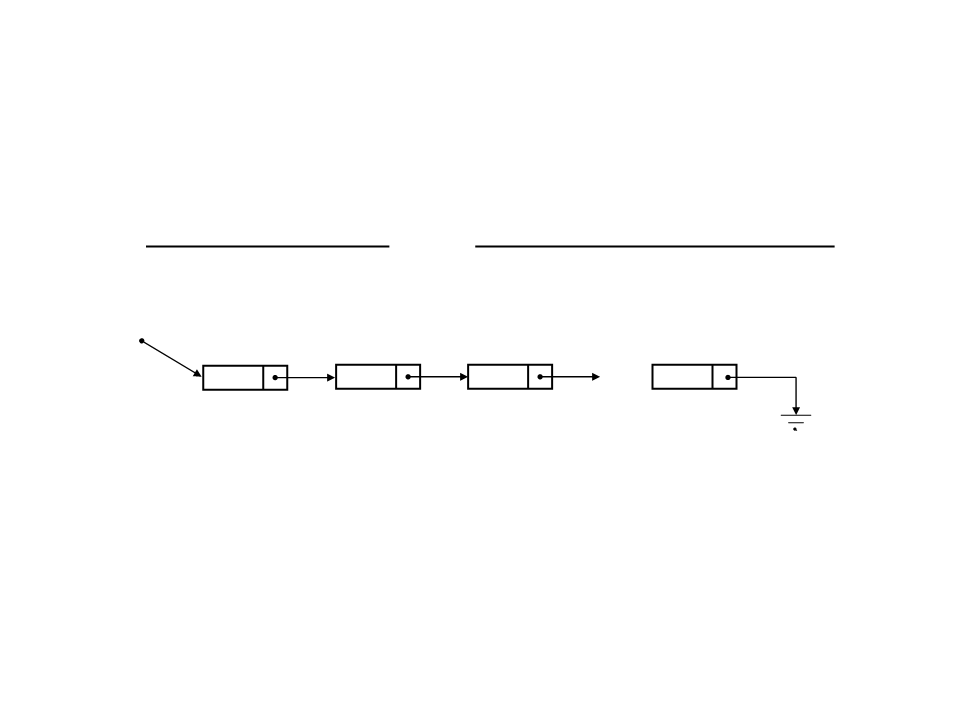A Linked List is a sequence of elements, where each element
has information stored and a pointer to the next element in
the sequence:
.
. .
DataN
Data1
Data2
Data3
Comprises a chained sequence of elements, called list nodes;
Each node is composed of two properties:
Stored data;
A pointer to the next element of the list;
The list is represented by a pointer to the first node;
The pointer of the last element is null.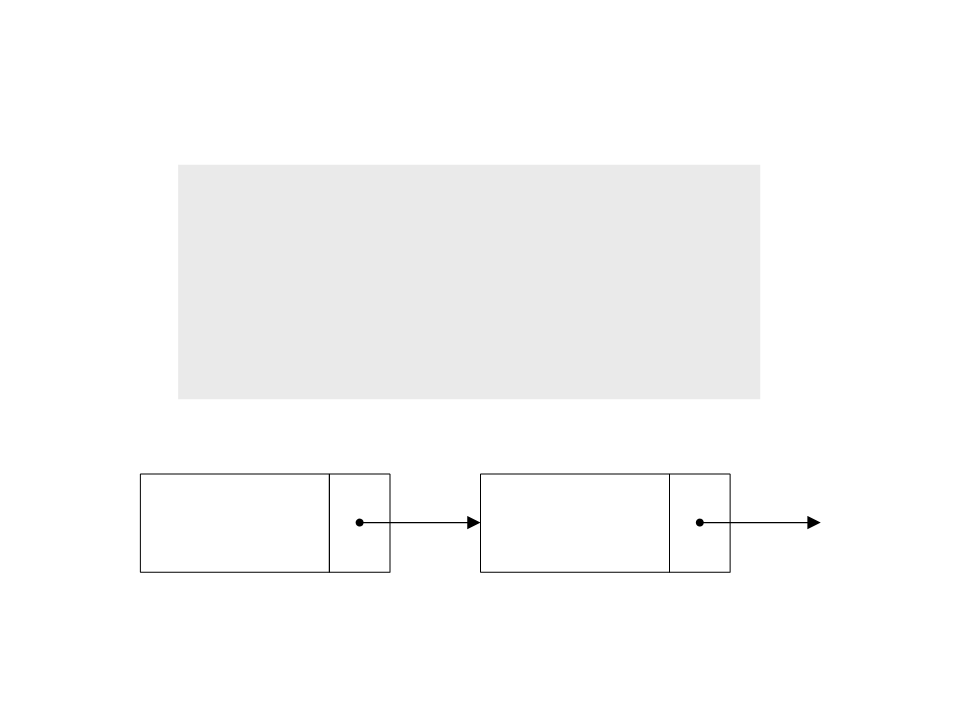public class Node<T>
{
private Node<T> next;
private T data;
.
..
}
next
next
data
data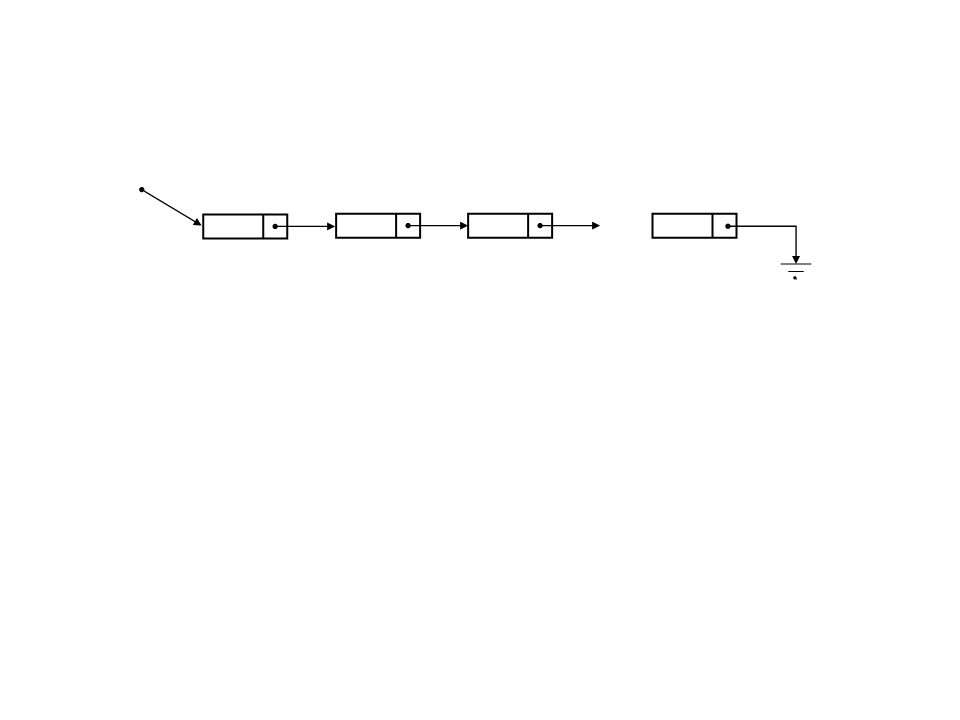.
. .
DataN
Data1
Data2
Data3
Create;
Insert;
Print;
Test empty;
Search;
Remove;
Sort;
Ordered insert;
Compare;
...public class Node<T>{
private Node<T> next;
private T data;
public Node(T t){
next = null;
data = t;
}
public Node<T> Next{
get { return next; }
set { next = value; }
}
public T Data{
get { return data; }
set { data = value; }
}
}
.
..
}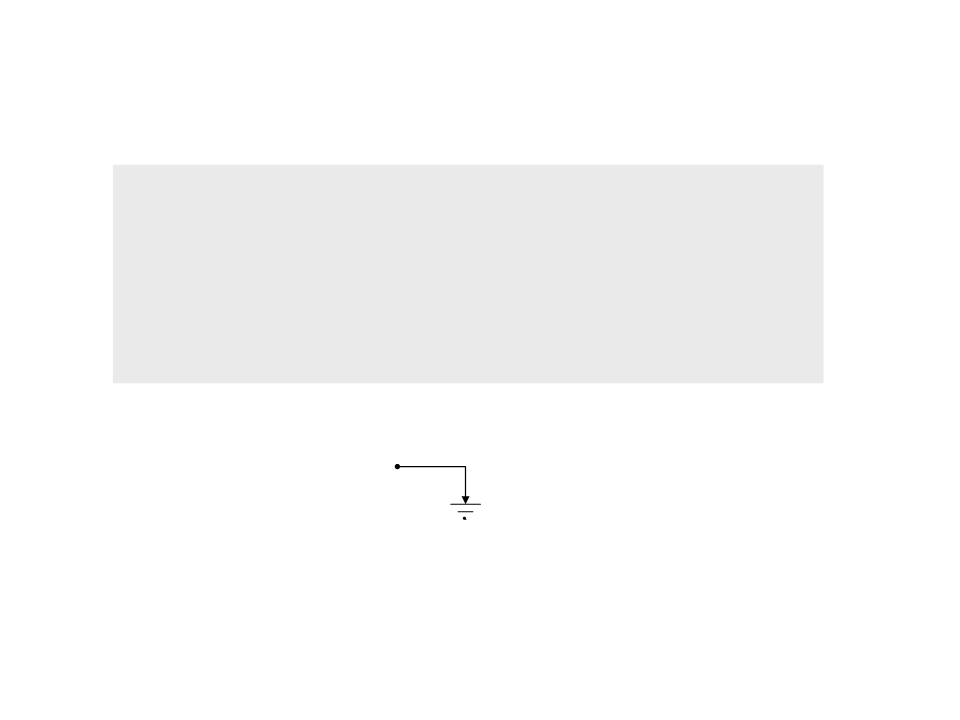O(1)
{
}
null
Creates a new empty linked list by pointing the head to null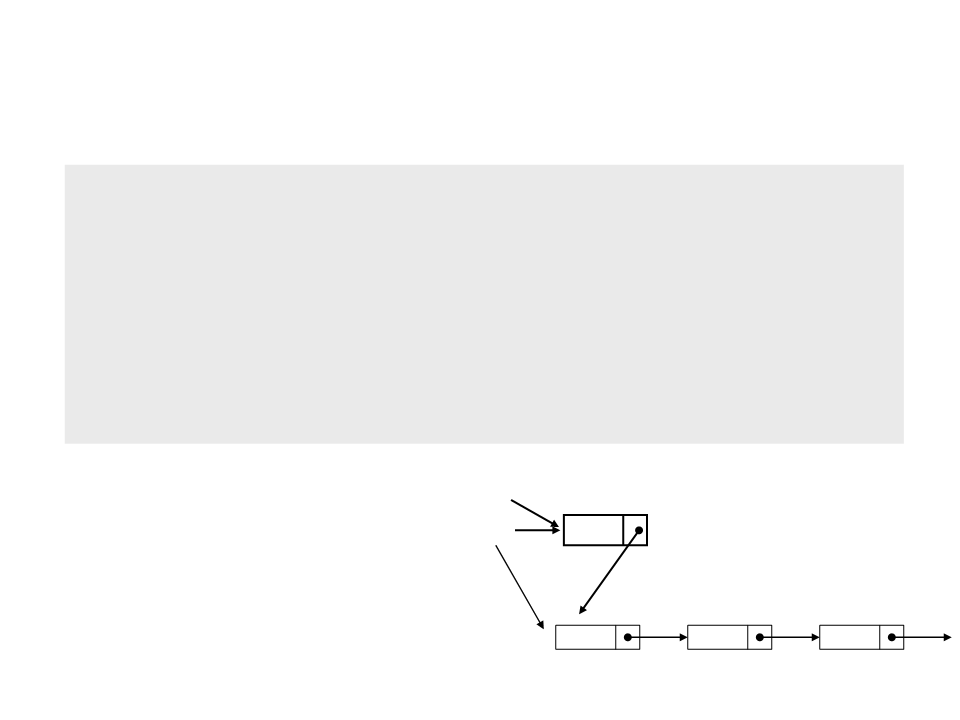Linked List Insert at the Beginning
O(1)
public void InsertAtBegin(T data)
{
Node<T> node = new Node<T>(data);
}
new node
1. Creates the new node;
data
2. Inserts the new node in
the beggining of the list;
Data1
Data2
Data3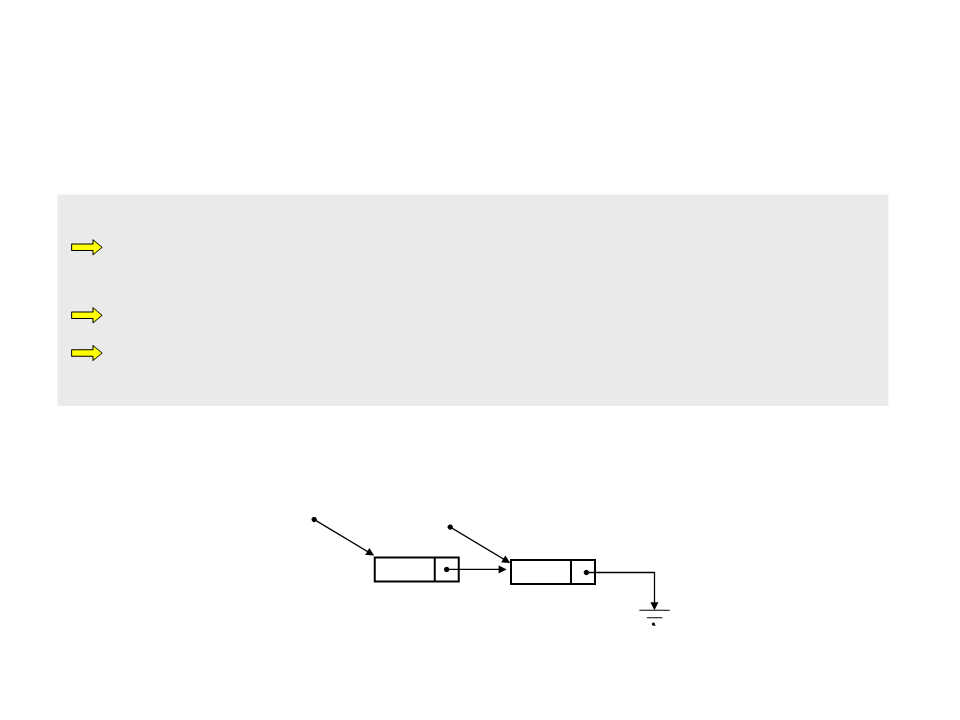myList.InsertAtBegin(23);
myList.InsertAtBegin(45);
45
23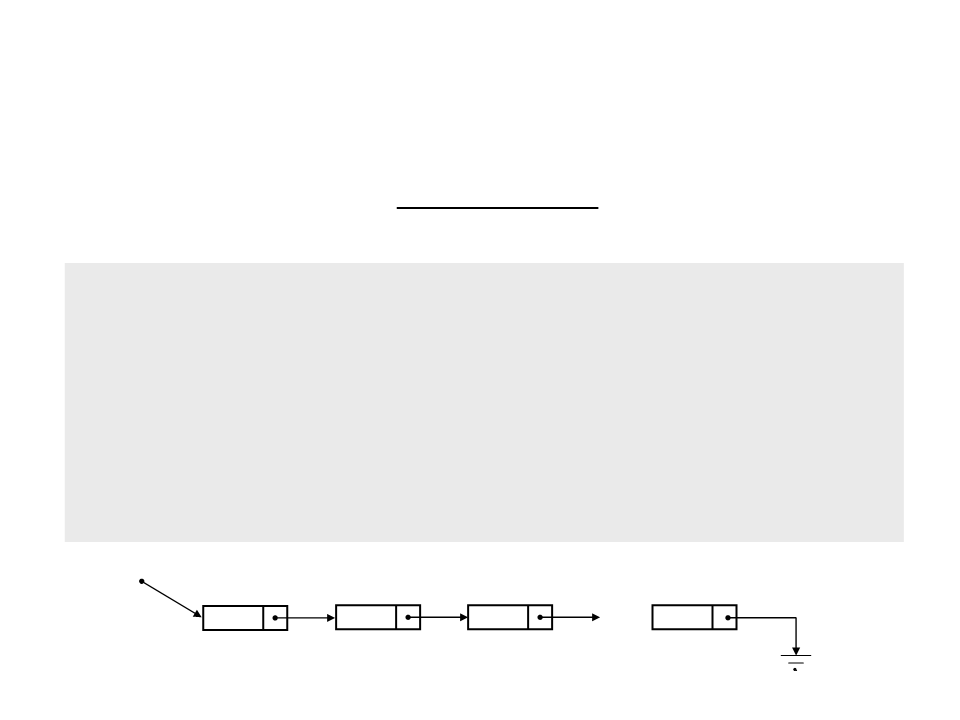We can implement the GetEnumerator method in the generic
class to allow us to iterate over the array using a foreach.
public IEnumerator<T> GetEnumerator()
O(n)
{
while (current != null)
{
yield return current.Data;
current = current.Next;
}
}
.
. .
DataN
Data1
Data2
Data3foreach (int i in myList)
{
Debug.Log(i);
}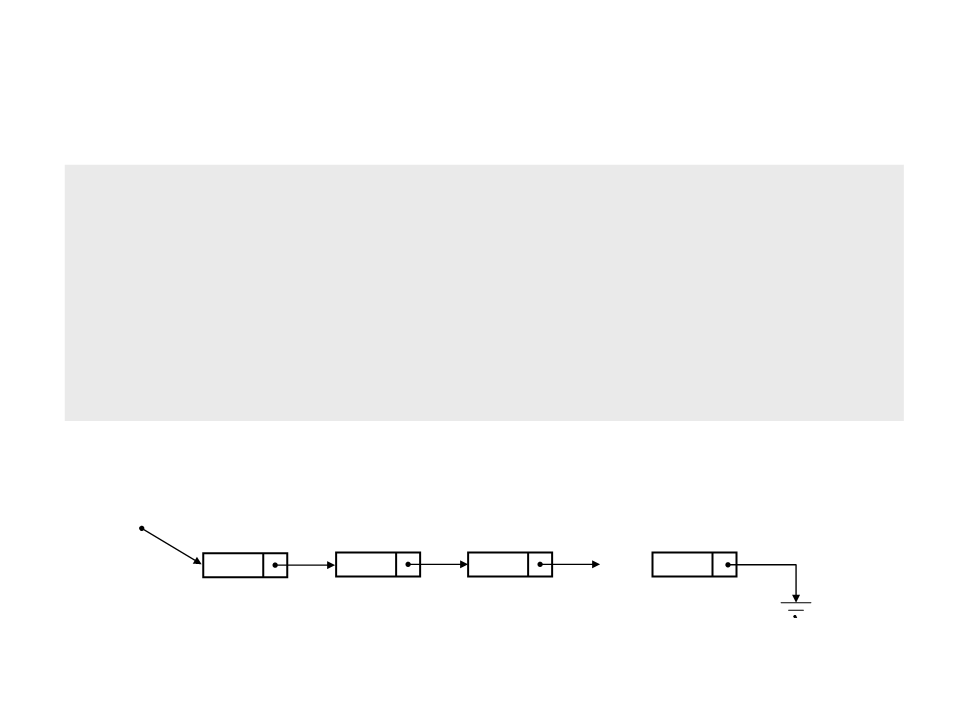public bool IsEmpty()
O(1)
{
return true;
else
return false;
}
.
. .
DataN
Data1
Data2
Data3public Node<T> Search(T data){
O(n)
while (current != null){
if (current.Data.Equals(data)){
return current;
}
current = current.Next;
}
return null;
}
Node<int> node = myList.Search(42);
if (node != null)
Debug.Log("Element found: " + node.Data);
elseLinked List Insert at the End
Two cases:
The new element will be the first element of the list;
The new element is not the first element of the list.
public void InsertAtEnd(T data){
O(n)
Node<T> node = new Node<T>(data);
}
else{
while (current.Next != null)
current = current.Next;
current.Next = node;
}
}public void InsertAfter(T after, T data)
O(n)
{
Node<T> afterNode = Search(after);
if (afterNode != null)
{
Node<T> newNode = new Node<T>(data);
Node<T> temp = afterNode.Next;
afterNode.Next = newNode;
newNode.Next = temp;
}
}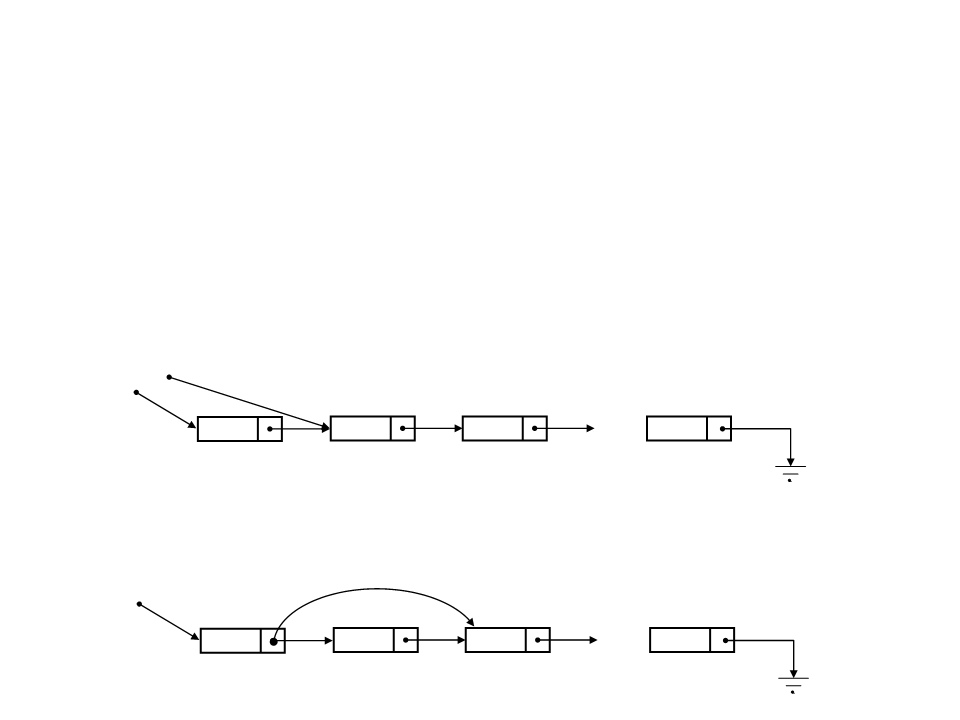Input: element to remove.
If the element to be removed is the first element of the list,
.
. .
DataN
Data1
Data2
Data3
Otherwise, remove the element and update the next pointer:
.
. .
DataN
Data1
Data2
Data3public void Remove(T data){
O(n)
Node<T> previous = null;
/
/search and keep both current and previous pointers
while ((current != null) && (!current.Data.Equals(data)))
{
previous = current;
current = current.Next;
}
if (current != null)
{
if (previous == null) //remove from the beginning
else
//remove from any other place
previous.Next = current.Next;
}
}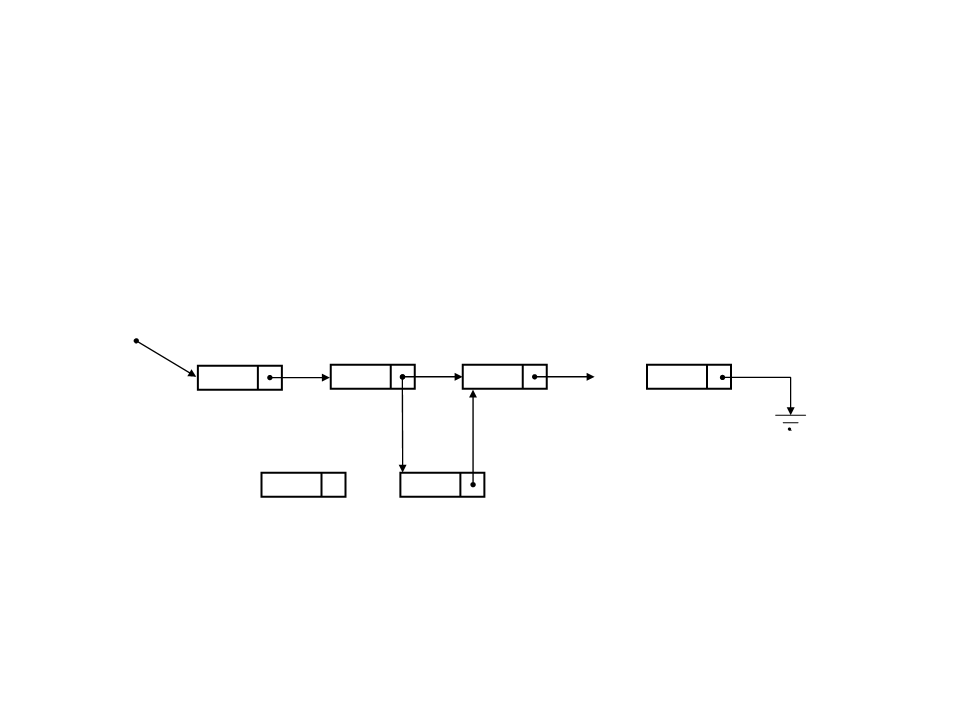The insert function iterates over the elements of the list until
it finds the correct position for inserting the new element:
.
. .
DataN
Data1
Data2
Data3
New
Newpublic void InsertOrdered(T data){
Node<T> newNode = new Node<T>(data);
Node<T> previous = null;
while ((current != null) && //search the correct position
(
current.Data.CompareTo(newNode.Data) < 0)){
previous = current;
current = current.Next;
}
if (previous == null){ //insert in the beginning
}
else{
newNode.Next = previous.Next;
previous.Next = newNode;
//insert in other places
}
}To compare data in a generic class using the CompareTo
function, the class must IComparable interface:
public class Node<T> where T: IComparable
{
.
..
}
public class LinkedList<T> where T : IComparable
{
.
..
}{
while ((current1 != null) && (current2 != null))
{
if (current1.Data.CompareTo(current2.Data) != 0)
return false;
current1 = current1.Next;
current2 = current2.Next;
}
/
/check if both lists ended at the same time
if ((current1 == null) && (current2 == null))
return true;
else
return false;
}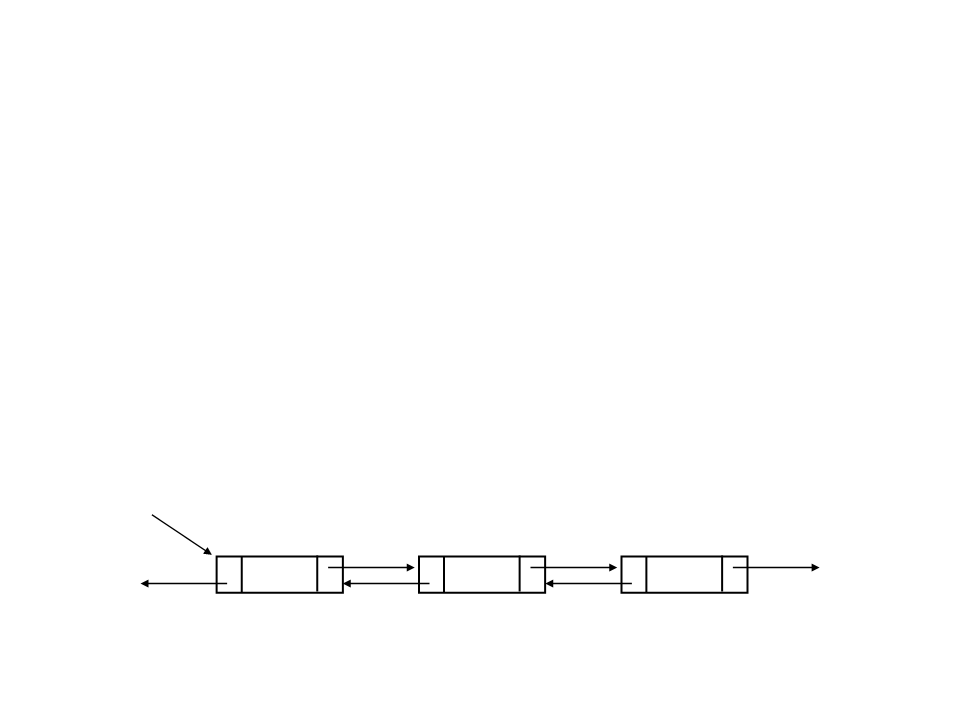Each element has a pointer to the next element and a pointer
to the previous element;
Given an element, it is possible to access the next and the
previous elements;
Given a pointer to the last element of the list, it is possible to
traverse over the list in reverse order;
Data1
Data2
Data3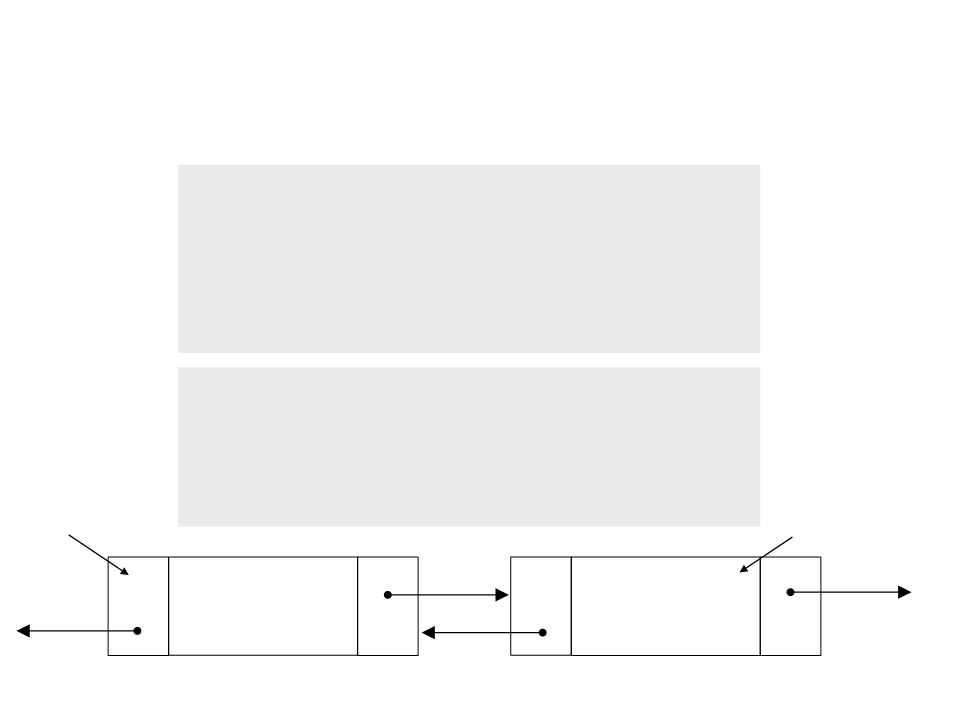public class DoublyNode<T>{
private DoublyNode<T> next;
private DoublyNode<T> prev;
private T data;
.
..
}
private DoublyNode<T> tail;
.
..
}
tail
next
next
data
data
prev
prev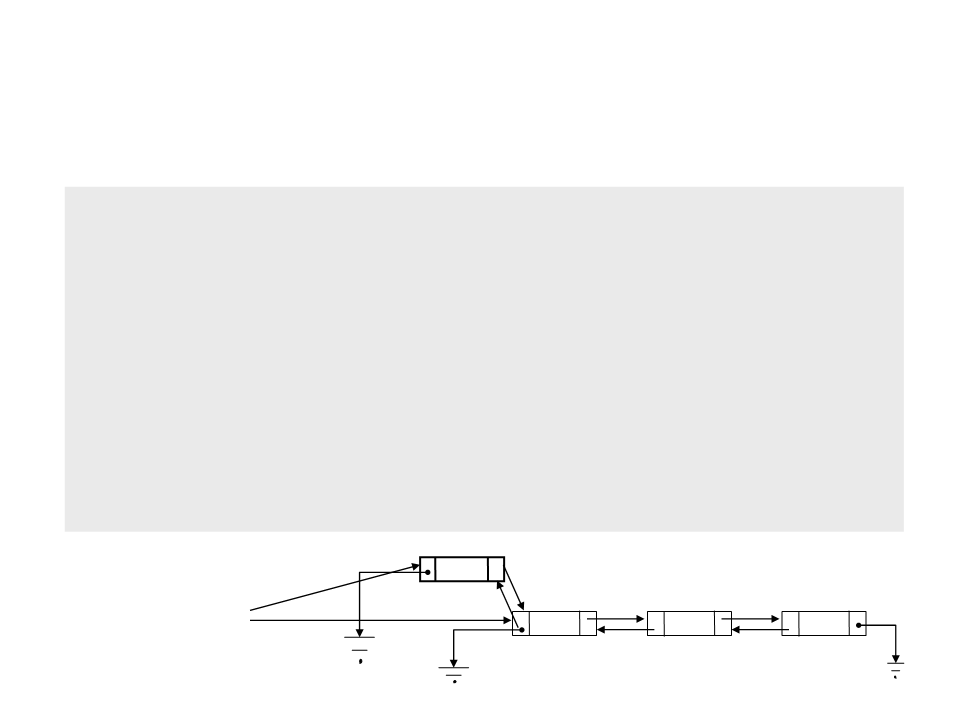Doubly Linked List Insert at the
Beginning
public void InsertAtBegin(T data){
DoublyNode<T> node = new DoublyNode<T>(data);
node.Data = data;
node.Prev = null;
if (tail == null)
tail = node;
}
New
Data1
Data2
Data3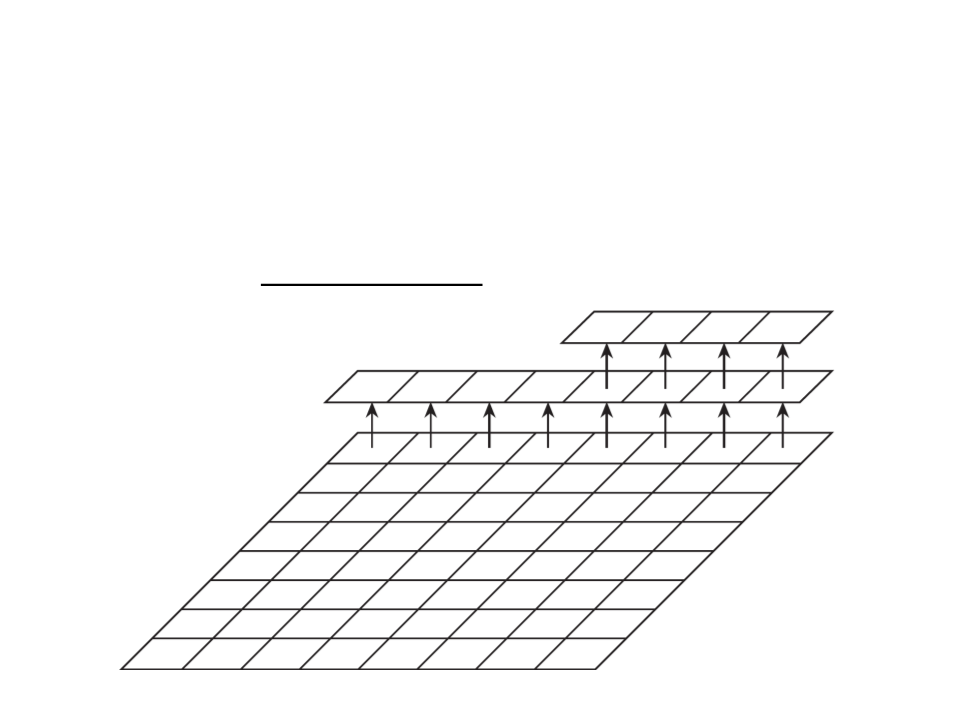Applications in Game Programming
Everywhere when dynamic set of objects is needed: list of
enemies, list of waypoints, list of bullets, player inventory,
game levels, layered tile maps, etc.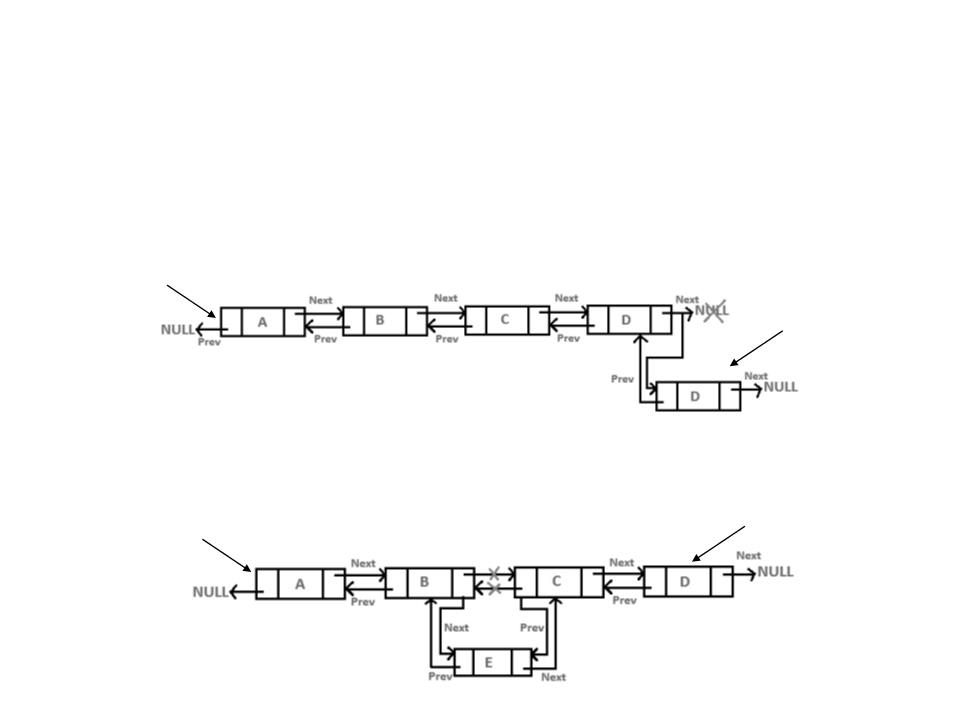1
) Implement a function to insert a new element at the end of a
tail
2
) Implement a function to insert a new element after an existing
element of a Doubly Linked List.
tail